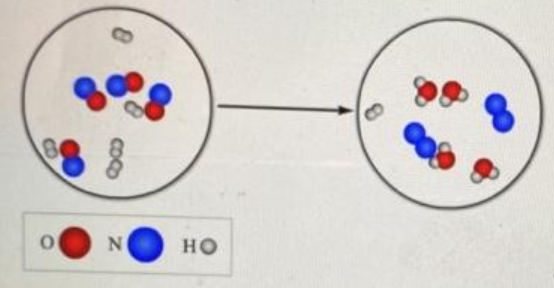# Problem: What is the chemical formula for the limiting reactant in the reaction shown?chemical formula: Write the balanced chemical equation for the reaction, using lowest whole number coefficientschemical equation:

###### FREE Expert Solution

For the first part, we're being asked to determine the chemical formula for the limiting reactant in the reaction shown.In the first scenario: NO and H2

90% (120 ratings)###### Problem DetailsWhat is the chemical formula for the limiting reactant in the reaction shown?

chemical formula:

Write the balanced chemical equation for the reaction, using lowest whole number coefficients

chemical equation:

Frequently Asked Questions

What scientific concept do you need to know in order to solve this problem?

Our tutors have indicated that to solve this problem you will need to apply the Limiting Reagent concept. You can view video lessons to learn Limiting Reagent. Or if you need more Limiting Reagent practice, you can also practice Limiting Reagent practice problems.